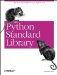# The Bastion Module

The Bastion module, shown in Example 14-7, allows you to control how a given object is used. It can be used to pass objects from unrestricted parts of your application to code running in restricted mode.

To create a restricted instance, simply call the Bastion wrapper. By default, all instance variables are hidden, as well as all methods that start with an underscore.

##### Example 14-7. Using the Bastion Module
```File: bastion-example-1.py

import Bastion

class Sample:
value = 0

def _set(self, value):
self.value = value

def setvalue(self, value):
if 10 < value <= 20:
self._set(value)
else:
raise ValueError, "illegal value"

def getvalue(self):
return self.value

#
# try it

s = Sample()
s._set(100) # cheat
print s.getvalue()

s = Bastion.Bastion(Sample())
s._set(100) # attempt to cheat
print s.getvalue()

100
Traceback (innermost last):
...
AttributeError: _set```

You can control which functions to publish. In Example 14-8, the internal method can be called from outside, but the getvalue no longer works.

##### Example 14-8. Using the Bastion Module with a Non-Standard Filter
```File: bastion-example-2.py

import Bastion

class Sample:
value = 0

def _set(self, value):
self.value = value

def setvalue(self, value):
if 10 < value <= 20:
self._set(value)
else:
raise ValueError, "illegal value"

def getvalue(self):
return self.value

#
# try it

def is_public(name):
return name[:3] != "get"

s = Bastion.Bastion(Sample(), is_public)
s._set(100) # this works
print s.getvalue() # but not this

100
Traceback (innermost last):
...
AttributeError: getvalue```Python Standard Library (Nutshell Handbooks) with
ISBN: 0596000960
EAN: 2147483647
Year: 2000
Pages: 252
Authors: Fredrik Lundh